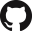# 30.101 Systems & Control¶

No. of Credits: 12 Subject Credits

Pre-requisites:

## Goal¶

The goal of this course is to introduce students to signal processing methods and control concepts used to model, analyze and design engineering systems. The course focuses on continuous linear time invariant (LTI) systems.

## Learning Objectives¶

• Demonstrate understanding of mathematical models by deriving transfer functions and state-space models of various physical systems (translational and rotational mechanical, electrical, electromechanical, fluid and thermal systems).
• Appreciate analogous systems where a model describing one physical system can be directly applied to an analogous system in another field.
• Understand the method to analyze, characterize and solve differential equations representing LTI systems using Laplace and inverse Laplace transforms.
• Analyze and simulate transient, steady-state and frequency response of first and second-order LTI systems.
• Understand the concept of characteristic equation, the notion of stability and the implication of stability in physical systems.
• Demonstrate understanding of full-state feedback control design using the pole placement technique.

## Measurable Outcomes¶

• Generate a representative mathematical model (transfer function and state space model) of a physical system. Describe features (system order, poles, zeros) and characteristics (stability, transient and steady-state response) of a physical system.
• Take advantage of mechanical-electrical analogies to derive the differential equations of mechanical systems using electrical RLC (resistor-inductor-capacitor) networks and vice versa using mass-spring-damper systems.
• Solve initial value problems using the Laplace transform method.
• Based on a performance specification (stability, settling time, disturbance rejection, steady state error), design a proportional-integral-differential (PID) feedback controller.
• Use the Routh’s stability criterion to assess the conditions necessary for stability in LTI systems.
• Being able to design feedback controllers for linear time invariant systems using the pole placement technique.

## Pedagogy¶

Integrated and unified theoretical and practical approach in signal processing and control engineering and their applications.

## Text & References¶

### Text¶

1. Feedback Control of Dynamic Systems by Gene F. Franklin, J. David Powell, Abbas Emami-Naeini
2. Modern Control Engineering by Katsuhiko Ogata
1. Signals and Systems by Alan V. Oppenheim, Alan S. Willsky with S. Hamid
2. Automatic Control Systems by Benjamin C. Kuo, Farid Golnaraghi

• Final exam (30%)
• Mid-term (25%)
• 1-D and 2-D Projects (15%)
• In-Class Quizzes & Homework (20%)
• Instructor prerogative (10%)

## Policies¶

• Full attendance is required.
• All assignments should be turned in on time.
• 1D and 2D projects are collaborative assignments. While groups may discuss over the approach and methodology, each group must submit a unique and independently prepared report.

## Course Notes¶

This set of course notes was graciously shared by Wei Min Cher, updated as of 26 April 2020.